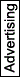# calculer translation | French-English dictionary

Search also in: Web News Encyclopedia Images Context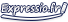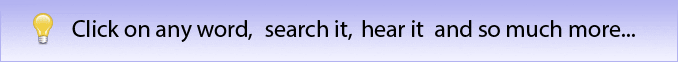## calculer

vt
(en comptant)
[+prix, total]
to calculate, to work out
J'ai calculé combien ça allait coûter.      I calculated how much it was going to cost., I worked out how much it was going to cost.
calculer qch de tête      to work sth out in one's head
(en estimant, évaluant)
[+impact, importance, effet]
to weigh up
calculer que      to work out that, to calculate that
→ j'ai calculé que ça prendrait plus de temps que prévu
(tactiquement)
[+action, réplique]
to calculate
Chaque geste est calculé.      Each move is calculated.
calculer son coup      to plan one's move
calculer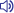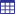vt.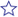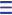calculate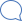se calculerv. be reckoned[Tech.]exp. to calculate thatexp. to work out thato. time it wellexp. to plan one's moveexp. to do a mental calculation of sthexp. to work sth out in one's headn. calculatorn. calculation [Tech.]n. stone [Med.]'calculer' also found in translations in English-French dictionary reckon upv. calculercomputev. calculer[Bus.]numeratev. calculerfigure outv. calculerconnumeratev. calculermiscalculatev. mal calculerexp. bien calculer qchprecomputev. calculer d'avance[Tech.]mistimev. mal calculer le tempsexp. calculer le moment propice